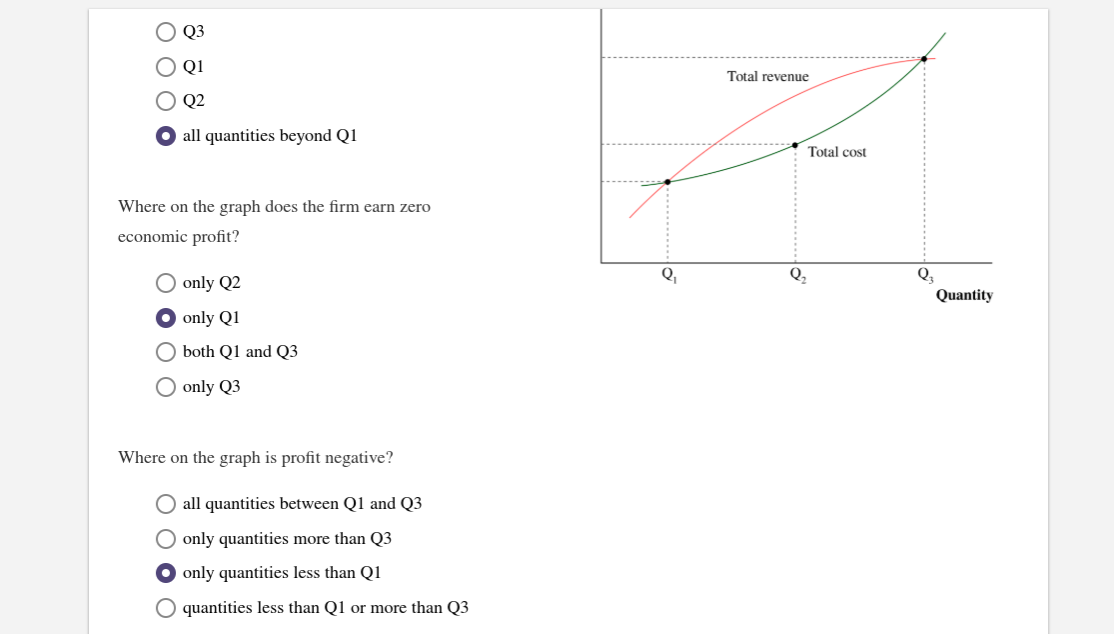# Q3O QiTotal revenueO Q2all quantities beyond Q1Total costWhere on the graph does the firm earn zeroeconomic profit?QQ2Q.QuantityO only Q2only Q1O both Q1 and Q3O only Q3Where on the graph is profit negative?O all quantities between Q1 and Q3O only quantities more than Q3O only quantities less than Q1quantities less than Q1 or more than Q3

Question
50 viewshelp_outlineImage TranscriptioncloseQ3 O Qi Total revenue O Q2 all quantities beyond Q1 Total cost Where on the graph does the firm earn zero economic profit? Q Q2 Q. Quantity O only Q2 only Q1 O both Q1 and Q3 O only Q3 Where on the graph is profit negative? O all quantities between Q1 and Q3 O only quantities more than Q3 O only quantities less than Q1 quantities less than Q1 or more than Q3 fullscreen
check_circle

Step 1

Since, the first question is not complete. So, I am solving second and third part.

Step 2

Zero-economic profit: Zero economic profit is also known as a normal profit. It refers to the condition where a firm’s total revenue is equal to its total cost. However, the point where “TR = TC” is also known as the break-even point.

Step 3

The zero-economic profit condition is, TR = TC. Thus, according to graph total revenue is equal to the tota...

### Want to see the full answer?

See Solution

#### Want to see this answer and more?

Solutions are written by subject experts who are available 24/7. Questions are typically answered within 1 hour.*

See Solution
*Response times may vary by subject and question.
Tagged in